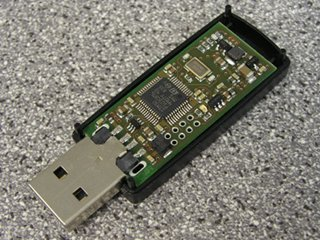Recent Events for foo.be MainPageDiary (Blog)

# TestingSimtecEntropyKey

When you are using /dev/random to generate OpenPGP public/private key pairs or other key materials for long-term, you might run into the common problems of lacking entropy in the pool. So you have to wait until enough entropy is gather on the system to continue the key generation. Especially gathering entropy on system might be difficult or even introduces some issues when the system is used by multiple users (http://www.pinkas.net/PAPERS/gpr06.pdf).

So I decided to acquire an external device to feed my entropy pool on my system. I looked at various solution and I finally found the Entropy Key from Simtec Electronics (http://www.entropykey.co.uk/tech/). The device is cleverly designed and use two noise generators as an entropy source. The output random stream is tested with the series of FIPS tests (http://www.entropykey.co.uk/res/download/diagram-explanation.pdf).## GNU/Linux System with Simtec entropy key installed

```% ekeydctl  list
NR,OK,Status,Path,SerialNo
4,YES,Running OK,/dev/entropykey/SERIALNUMBER,SERIALNUMBER

cat /proc/sys/kernel/random/entropy_avail
3968

dd if=/dev/random bs=1k count=100| ent
0+100 records in
0+100 records out
9531 bytes (9.5 kB) copied, 4.45569 s, 2.1 kB/s
Entropy = 7.982495 bits per byte.

Optimum compression would reduce the size
of this 9531 byte file by 0 percent.

Chi square distribution for 9531 samples is 230.82, and randomly
would exceed this value 75.00 percent of the times.

Arithmetic mean value of data bytes is 127.5063 (127.5 = random).
Monte Carlo value for Pi is 3.209068010 (error 2.15 percent).
Serial correlation coefficient is 0.002858 (totally uncorrelated = 0.0).
```

## Same without the entropy key

It took 3551.01 seconds without the entropy key compared to 4.4 seconds with the entropy key attached to get some random values.

```dd if=/dev/random bs=1k count=100| ent

0+100 records in
0+100 records out
805 bytes (805 B) copied, 3551.03 s, 0.0 kB/s
Entropy = 7.711860 bits per byte.

Optimum compression would reduce the size
of this 805 byte file by 3 percent.

Chi square distribution for 805 samples is 286.74, and randomly
would exceed this value 10.00 percent of the times.

Arithmetic mean value of data bytes is 123.2795 (127.5 = random).
Monte Carlo value for Pi is 3.194029851 (error 1.67 percent).
Serial correlation coefficient is -0.051766 (totally uncorrelated = 0.0).

```

## Are they any good alternative to an external hardware source?

You might want to have a look at Havege http://www.irisa.fr/caps/projects/hipsor/ that is relying on large set of small changes on the system. There is a daemon taking advantage of that algorithm http://www.issihosts.com/haveged/ and it seems to work quite well. Having an independent external source is not always possible, this seems to be a good alternative.